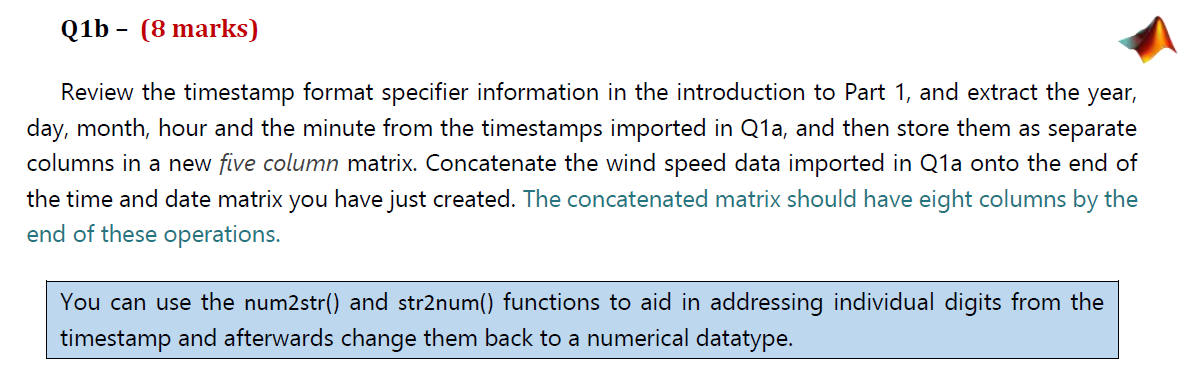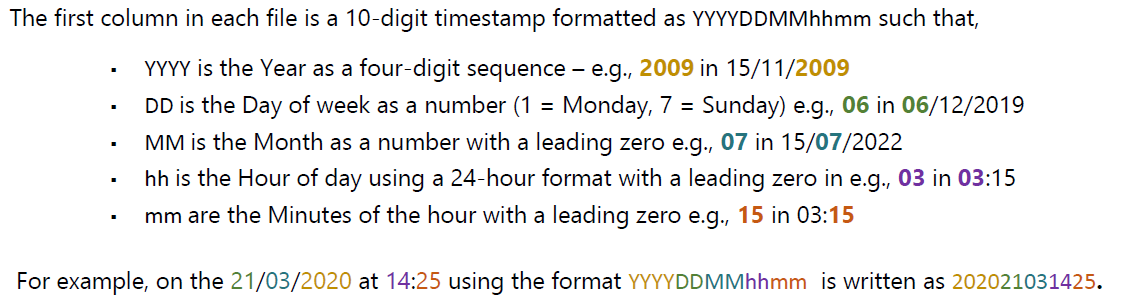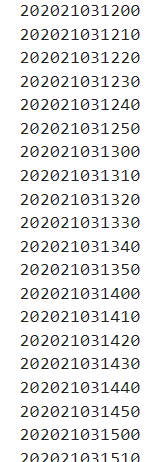Home / Expert Answers / Computer Science / matlab-coding-how-do-i-extract-the-year-date-month-hour-and-minute-nbsp-i-need-just-the-timestamp-pa182

# (Solved): MATLAB CODING HOW DO I EXTRACT THE YEAR DATE MONTH HOUR AND MINUTE  I NEED JUST THE TIMESTAMP ...

MATLAB CODING

HOW DO I EXTRACT THE YEAR DATE MONTH HOUR AND MINUTEI NEED JUST THE TIMESTAMP PART. THE TIMESTAMP IS IN THE FORMAT-TIMESTAMP IS A COLUMN MATRIX THAT HAS 5000 DATA VALUES AND LOOKS LIKE SUCHReview the timestamp format specifier information in the introduction to Part 1, and extract the year, day, month, hour and the minute from the timestamps imported in Q1a, and then store them as separate columns in a new five column matrix. Concatenate the wind speed data imported in Q1a onto the end of the time and date matrix you have just created. The concatenated matrix should have eight columns by the end of these operations. You can use the num2str() and str2num() functions to aid in addressing individual digits from the timestamp and afterwards change them back to a numerical datatype. The first column in each file is a 10-digit timestamp formatted as YYYYDDMMhhmm such that, - YYYY is the Year as a four-digit sequence - e.g., 2009 in 15/11/2009 - $$\mathrm{DD}$$ is the Day of week as a number ( $$1=$$ Monday, $$7=$$ Sunday) e.g., 06 in 06/12/2019 - MM is the Month as a number with a leading zero e.g., 07 in 15/07/2022 - hh is the Hour of day using a 24-hour format with a leading zero in e.g., 03 in 03:15 - $$\mathrm{mm}$$ are the Minutes of the hour with a leading zero e.g., 15 in 03:15 202021031200 202021031210 202021031220 202021031230 202021031240 202021031250 202021031300 202021031310 202021031320 202021031330 202021031340 202021031350 202021031400 202021031410 202021031420 202021031430 202021031440 202021031450 202021031500 220210215

We have an Answer from Expert